qplot() is now deprecated in order to encourage the users to learn ggplot() as it makes it easier to create complex graphics.

## Usage

qplot(
x,
y,
...,
data,
facets = NULL,
margins = FALSE,
geom = "auto",
xlim = c(NA, NA),
ylim = c(NA, NA),
log = "",
main = NULL,
xlab = NULL,
ylab = NULL,
asp = NA,
stat = deprecated(),
position = deprecated()
)

quickplot(
x,
y,
...,
data,
facets = NULL,
margins = FALSE,
geom = "auto",
xlim = c(NA, NA),
ylim = c(NA, NA),
log = "",
main = NULL,
xlab = NULL,
ylab = NULL,
asp = NA,
stat = deprecated(),
position = deprecated()
)

## Arguments

x, y, ...

Aesthetics passed into each layer

data

Data frame to use (optional). If not specified, will create one, extracting vectors from the current environment.

facets

faceting formula to use. Picks facet_wrap() or facet_grid() depending on whether the formula is one- or two-sided

margins

See facet_grid(): display marginal facets?

geom

Character vector specifying geom(s) to draw. Defaults to "point" if x and y are specified, and "histogram" if only x is specified.

xlim, ylim

X and y axis limits

log

Which variables to log transform ("x", "y", or "xy")

main, xlab, ylab

Character vector (or expression) giving plot title, x axis label, and y axis label respectively.

asp

The y/x aspect ratio

stat, position## Examples

# Use data from data.frame
qplot(mpg, wt, data = mtcars)
#> Warning: qplot() was deprecated in ggplot2 3.4.0.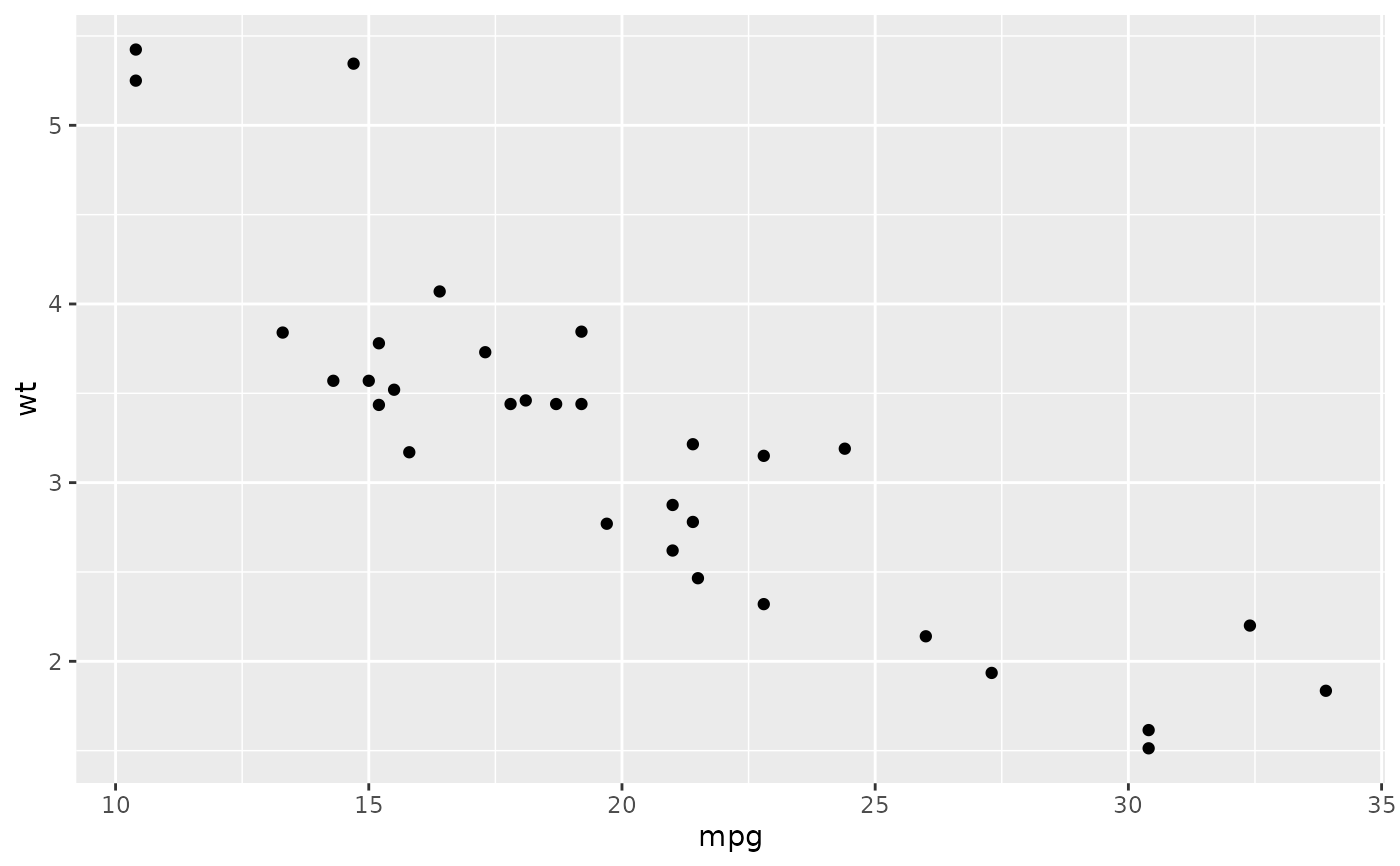qplot(mpg, wt, data = mtcars, colour = cyl)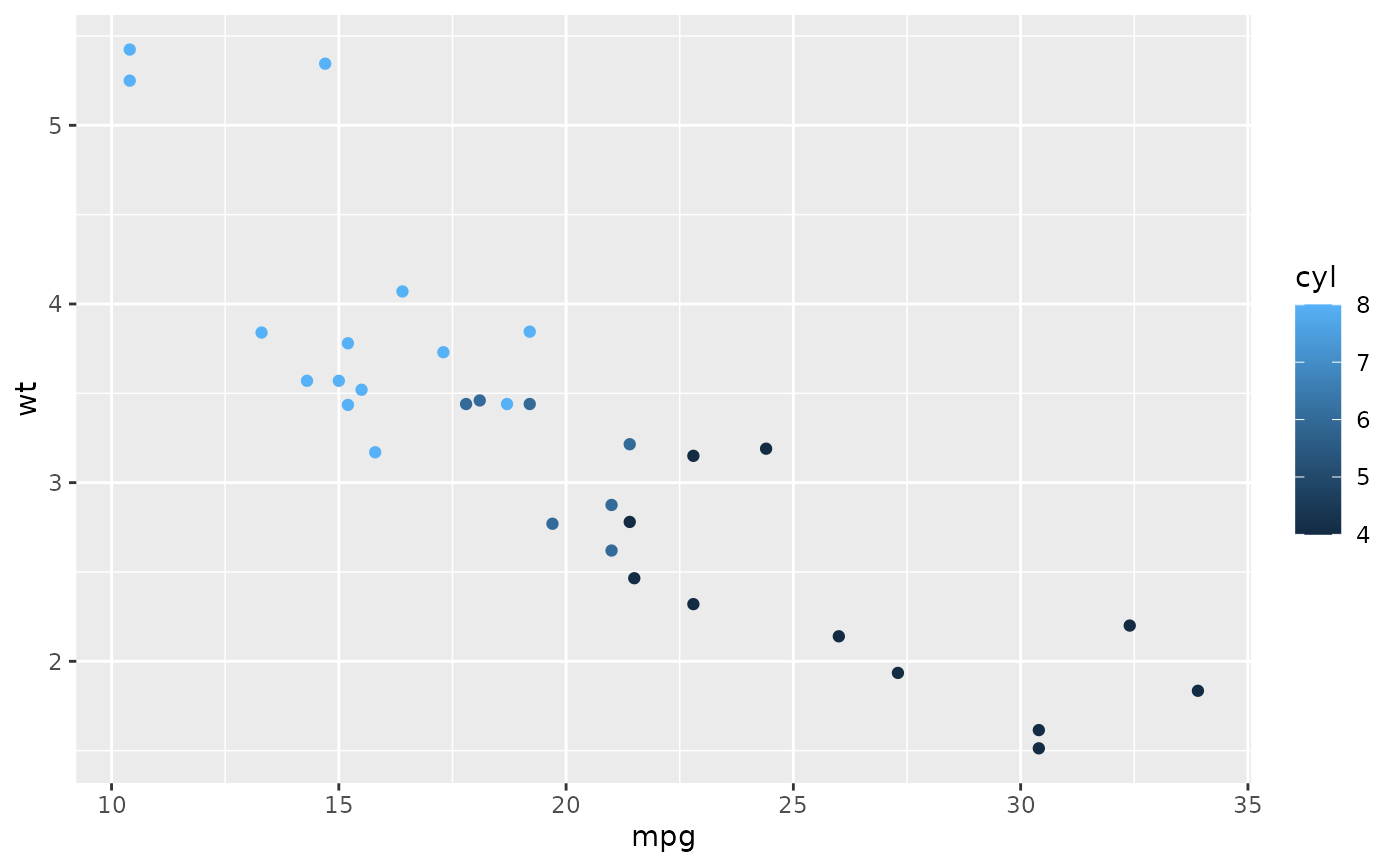qplot(mpg, wt, data = mtcars, size = cyl)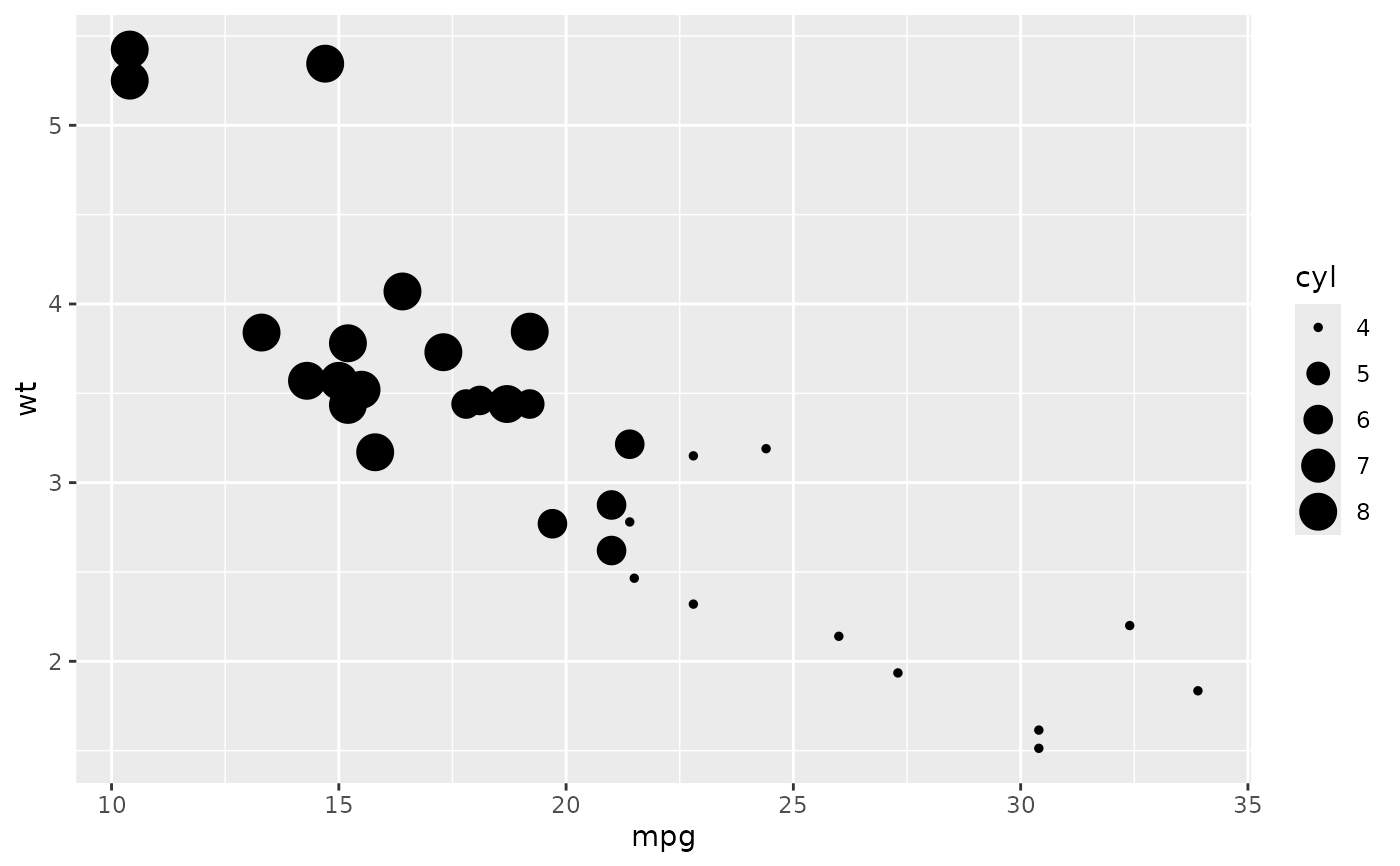qplot(mpg, wt, data = mtcars, facets = vs ~ am)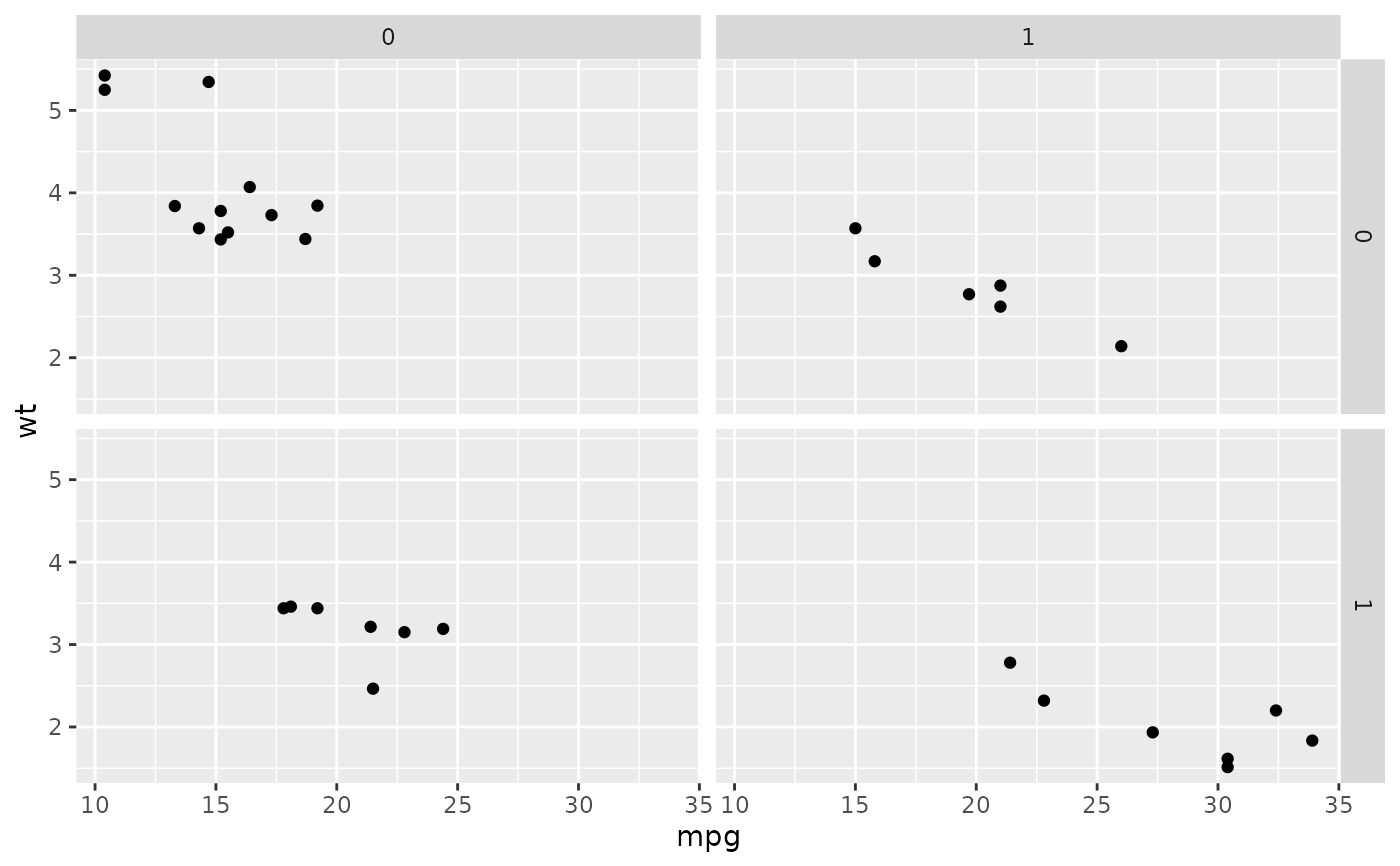# \donttest{
set.seed(1)
qplot(1:10, rnorm(10), colour = runif(10))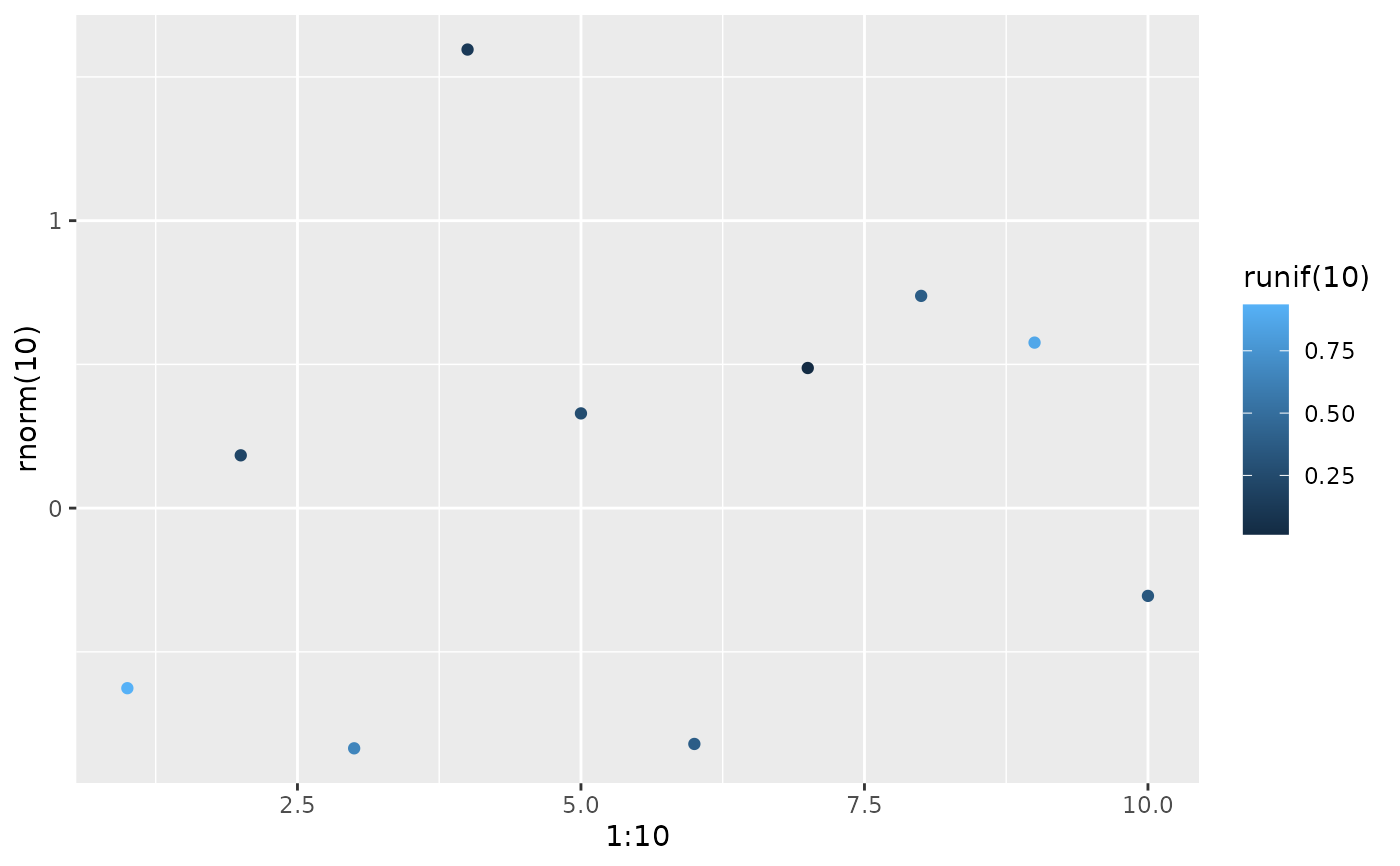qplot(1:10, letters[1:10])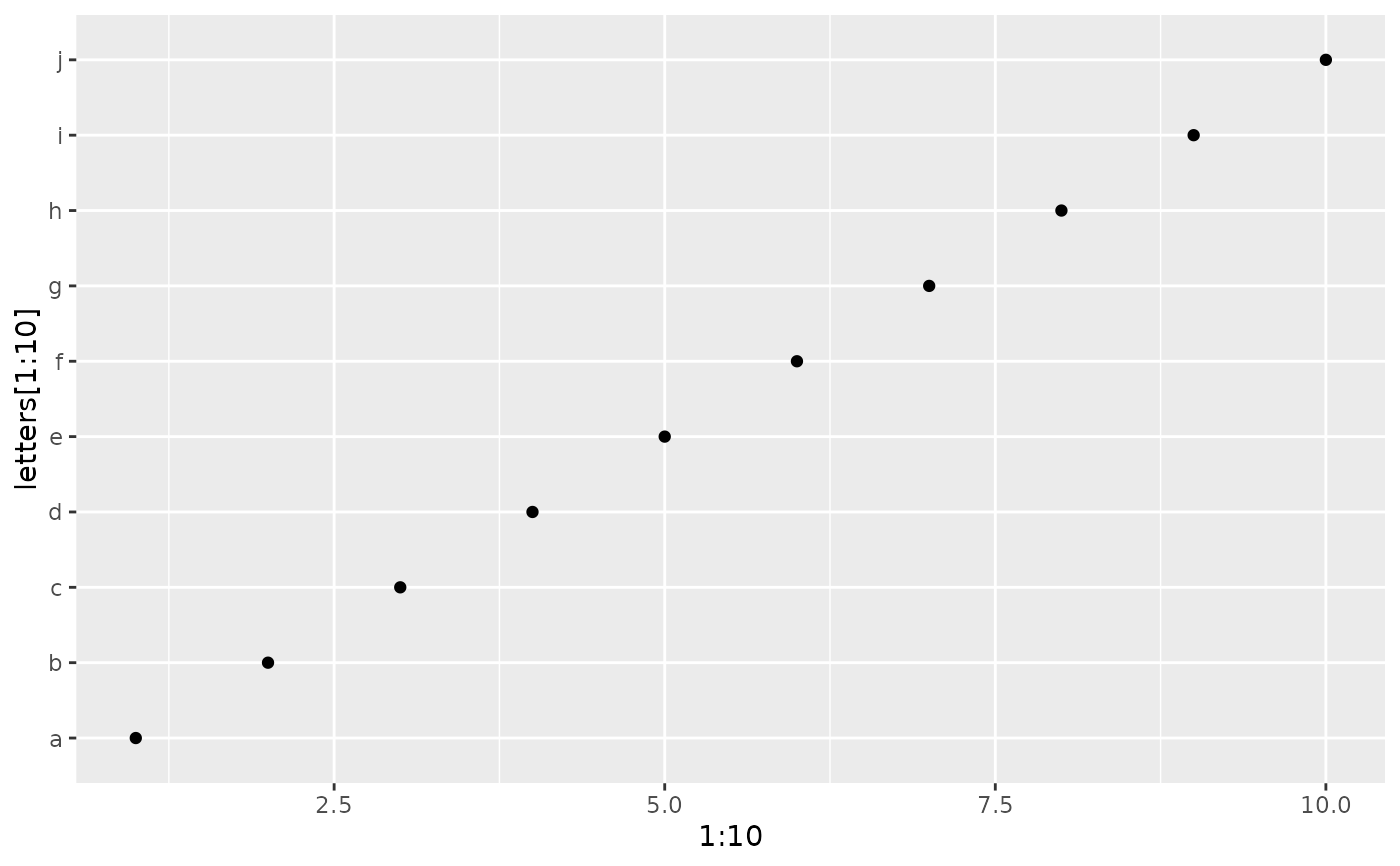mod <- lm(mpg ~ wt, data = mtcars)
qplot(resid(mod), fitted(mod))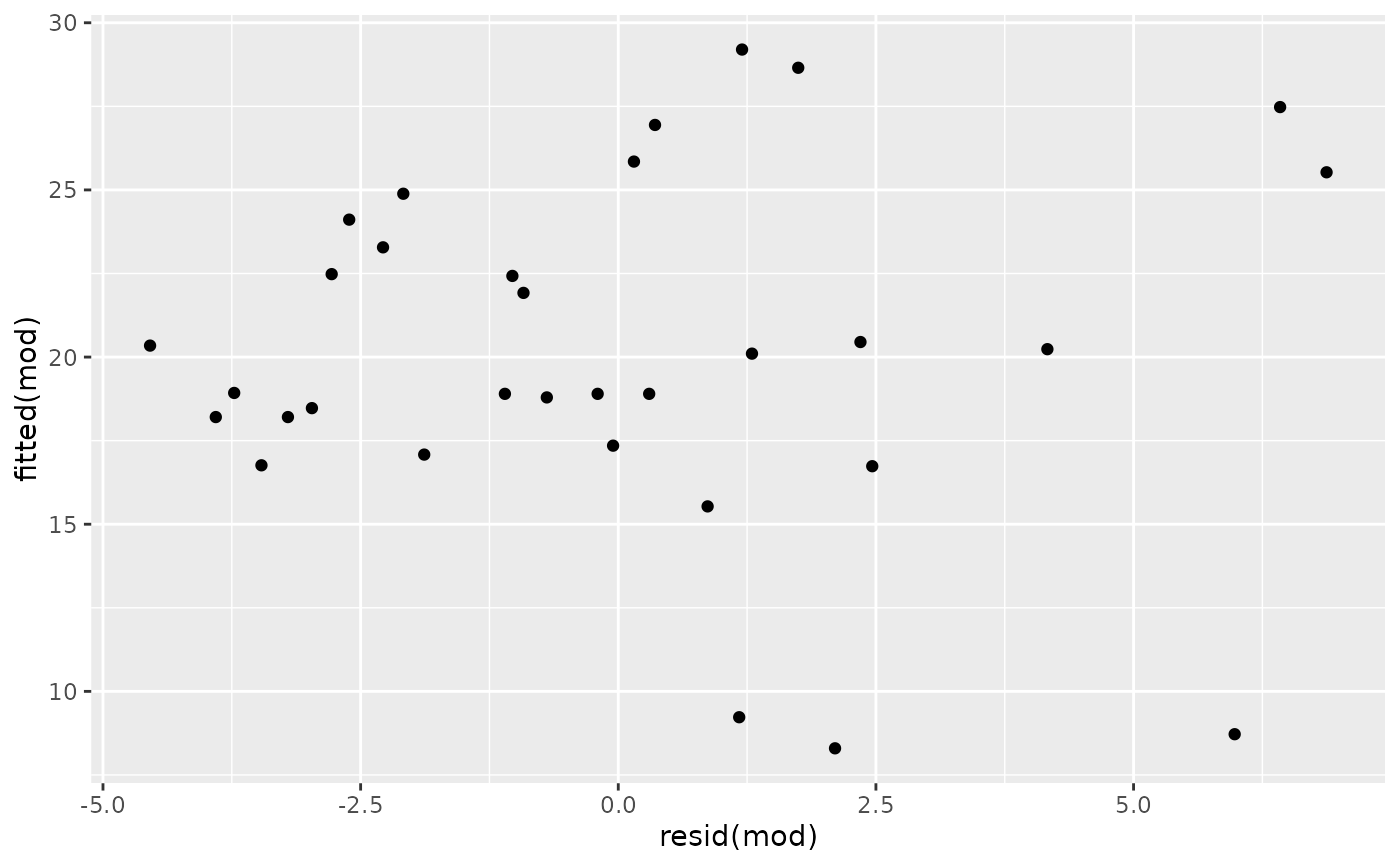f <- function() {
a <- 1:10
b <- a ^ 2
qplot(a, b)
}
f()# To set aesthetics, wrap in I()
qplot(mpg, wt, data = mtcars, colour = I("red"))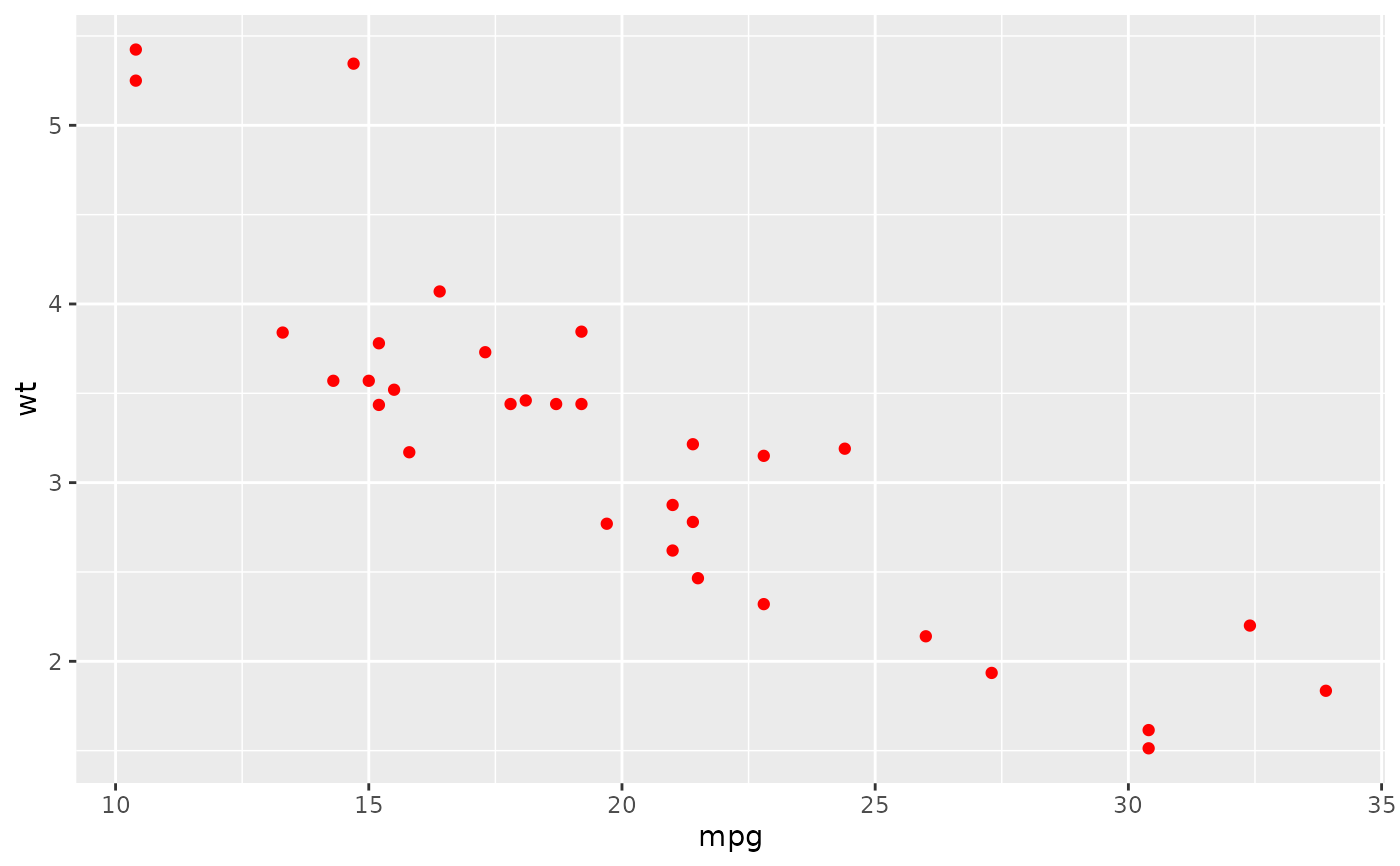# qplot will attempt to guess what geom you want depending on the input
# both x and y supplied = scatterplot
qplot(mpg, wt, data = mtcars)# just x supplied = histogram
qplot(mpg, data = mtcars)
#> stat_bin() using bins = 30. Pick better value with binwidth.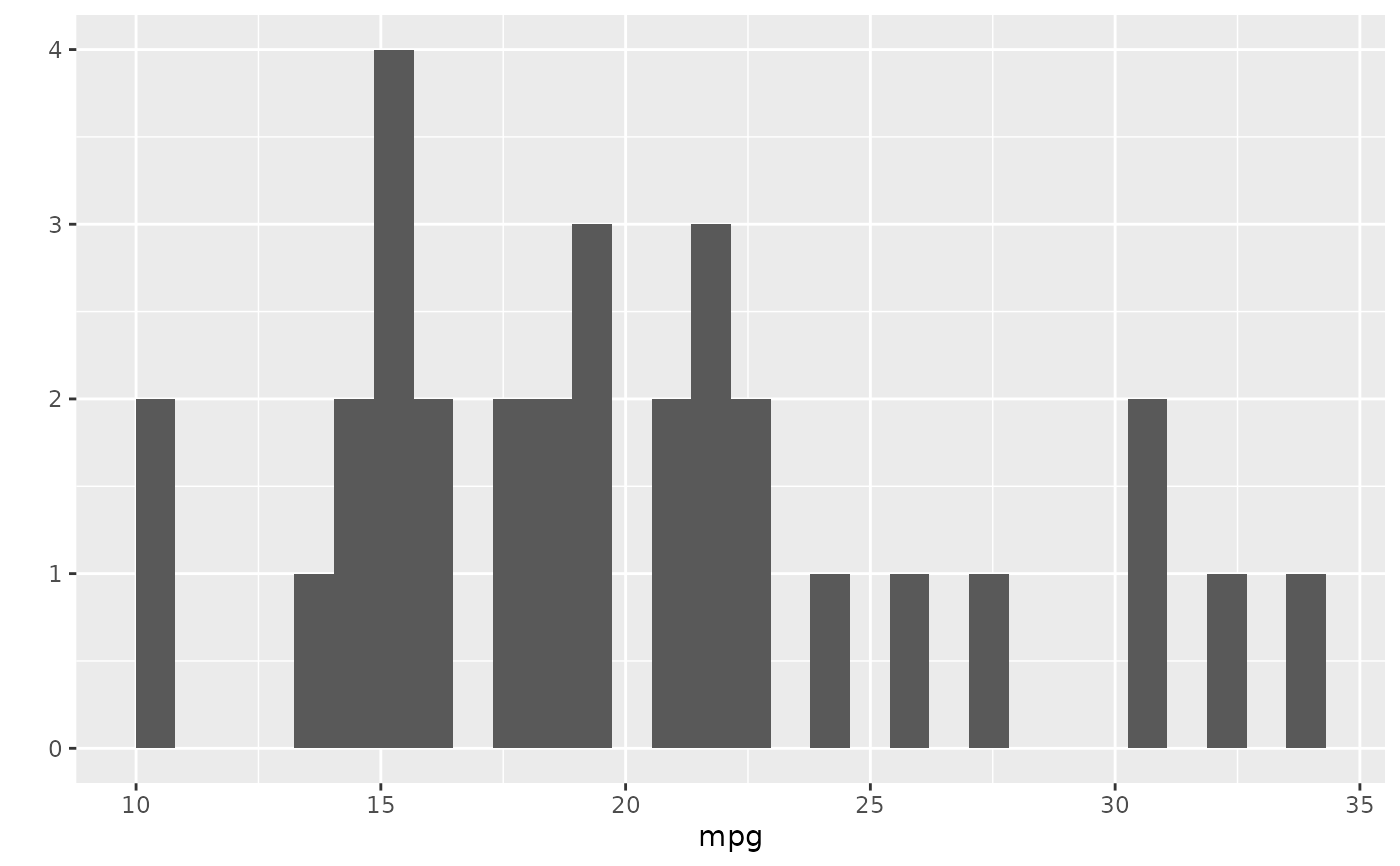# just y supplied = scatterplot, with x = seq_along(y)
qplot(y = mpg, data = mtcars)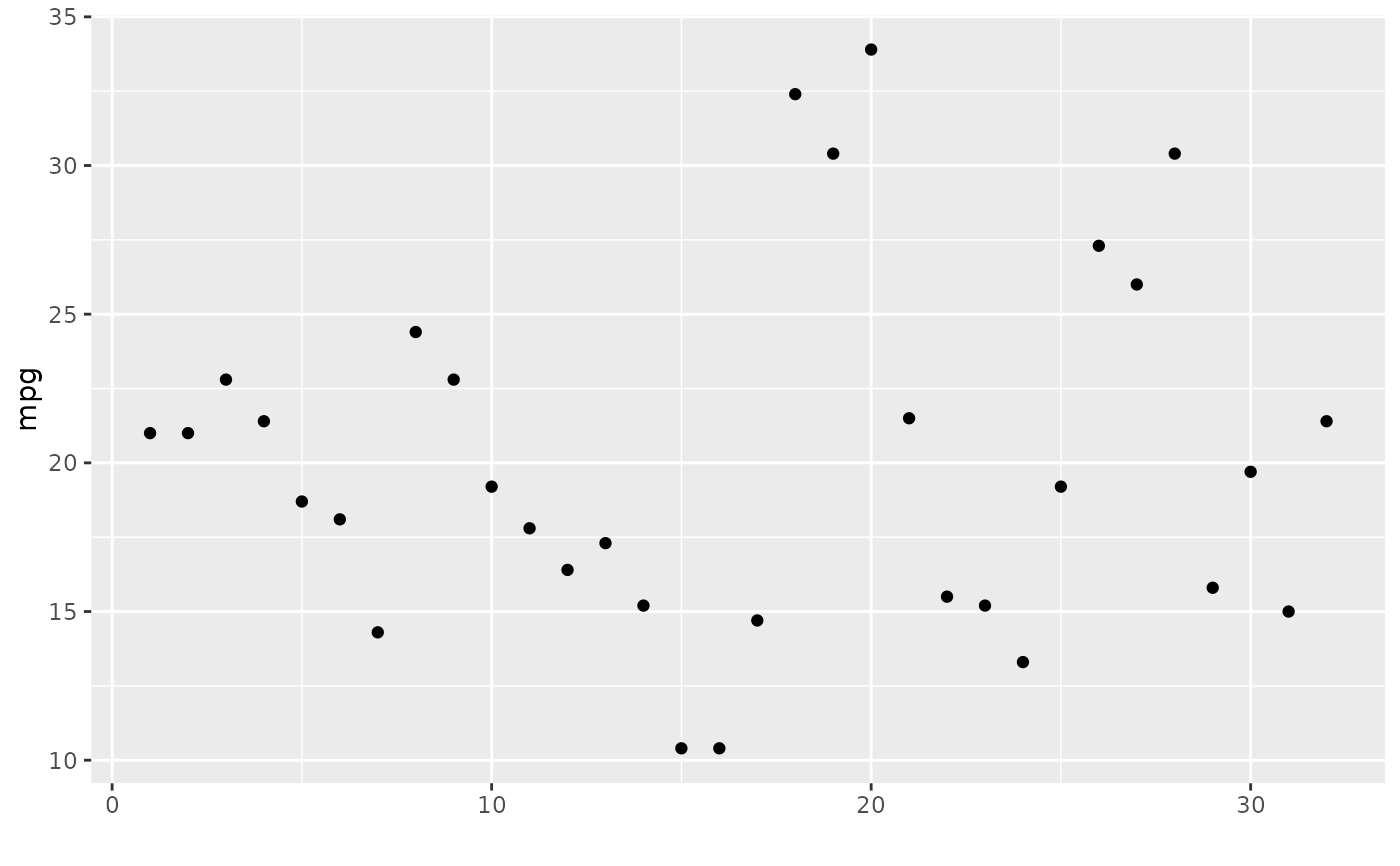# Use different geoms
qplot(mpg, wt, data = mtcars, geom = "path")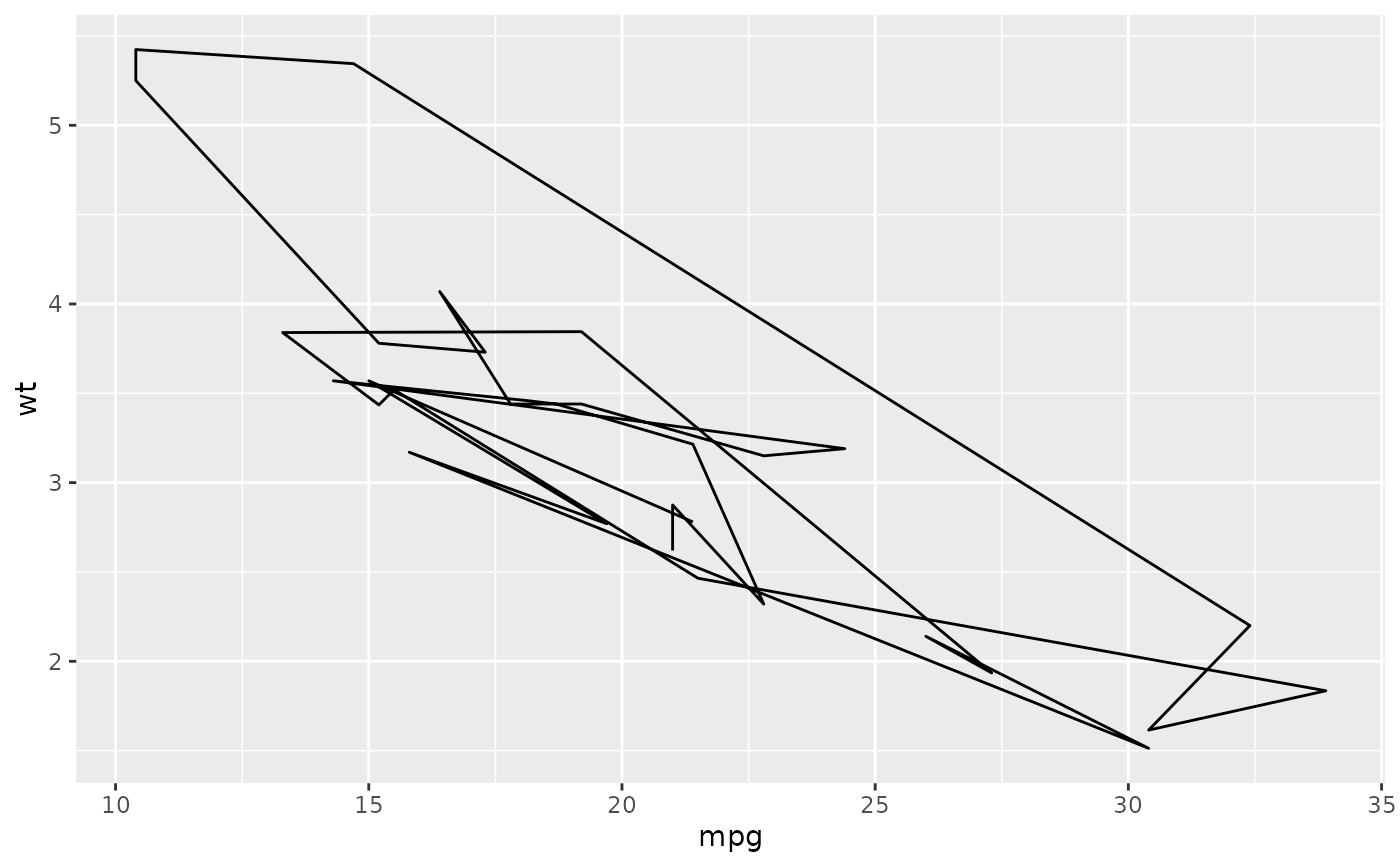qplot(factor(cyl), wt, data = mtcars, geom = c("boxplot", "jitter"))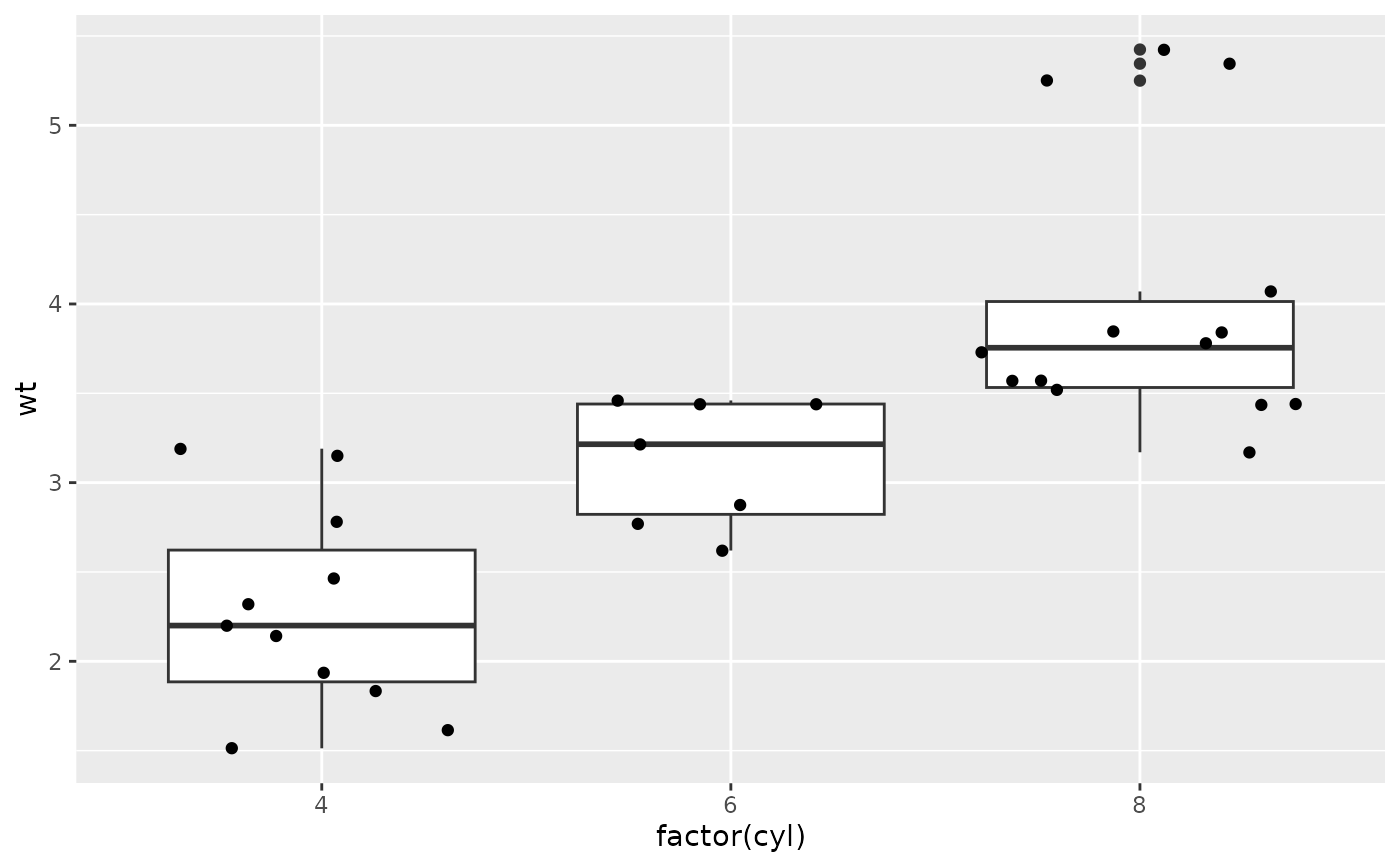qplot(mpg, data = mtcars, geom = "dotplot")
#> Bin width defaults to 1/30 of the range of the data. Pick better value
#> with binwidth.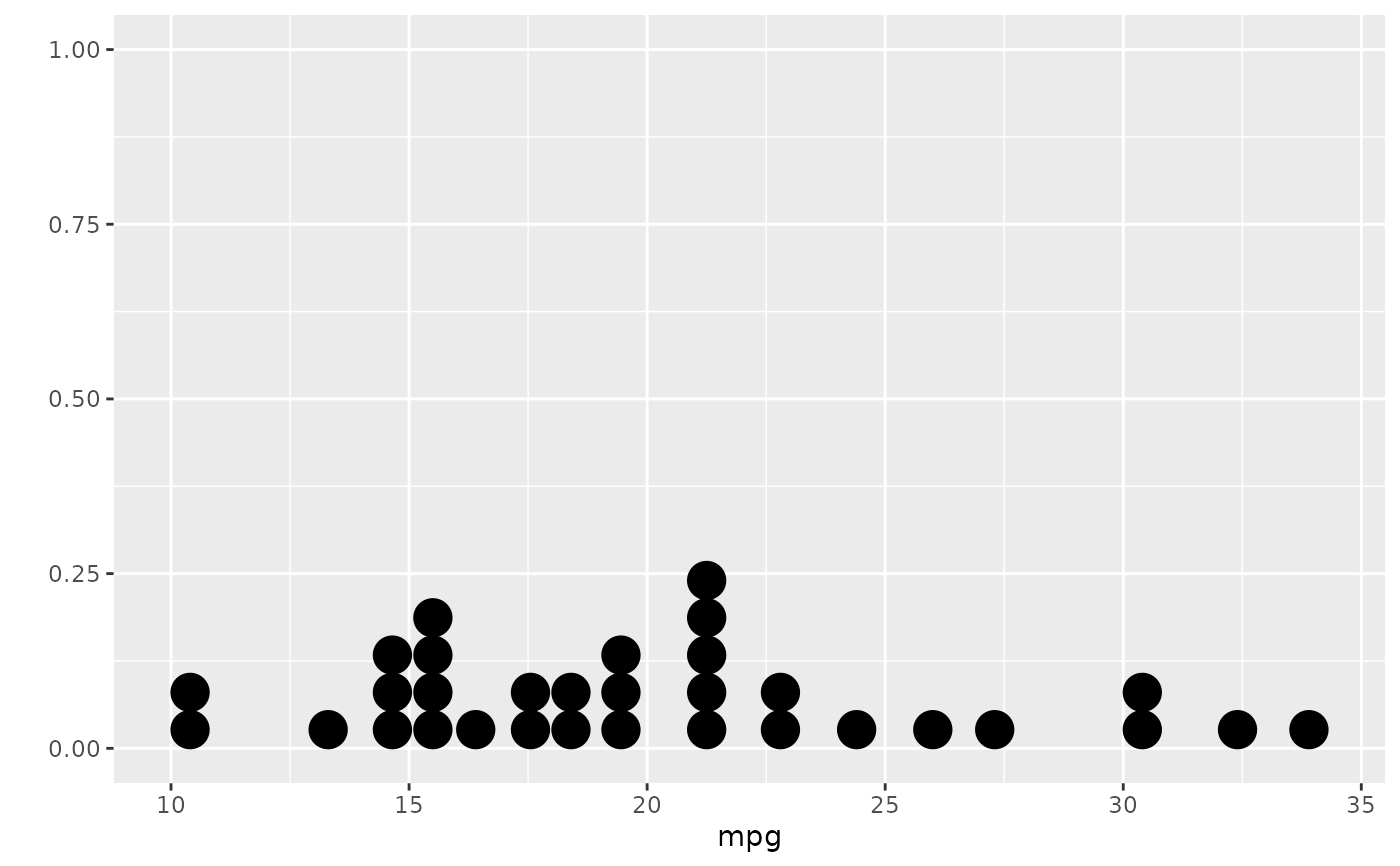# }